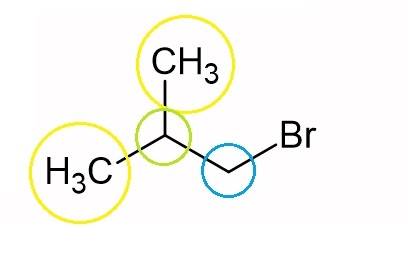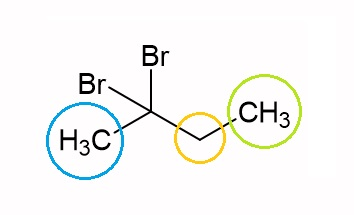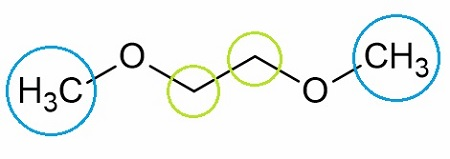# Draw a compound that is consistent with the following ^3H NMR data: a. C_4H_9Br, has 3 signals...

## Question:

Draw a compound that is consistent with the following ^3H NMR data:

a. C_4H_9Br, has 3 signals in the ^1H NMR spectrum, 2 doublets and a 9-line multiplet.

b. C_4H_9Br_2, has 3 signals in ^1H NMR spectrum, a singlet, a triplet and a quartet.

Give the structure of a compound with a formula of C_4H_10O_2 that gives only two singlets in the ^1H NMR spectrum in an integral ratio of 3:2.

## HNMR Spectroscopy:

NMR is widely used in elucidation of chemical structures. Proton NMR or H NMR is sensitive to the hydrogen nuclei in a substance which is why it is a good option for analysis of hydrogen-containing organic compounds.

a. {eq}C_4H_9Br {/eq}Hydrogen atoms encircled yellow will give a doublet, green has a 9-line multiplet and blue has a doublet.

b. {eq}C_4H_8Br_2 {/eq}The hydrogen atoms encircled blue is an NMR singlet, yellow is a quartet, and green is a triplet.

c. {eq}C_4H_{10}O_2 {/eq}The hydrogen atoms encircled blue and green give an integral ratio of 3:2.How to Read NMR Spectra of Organic Compounds

from Organic Chemistry: Help & Review

Chapter 2 / Lesson 2
17K

Support